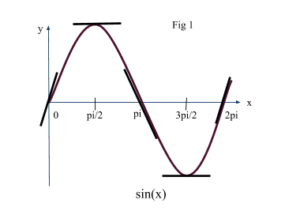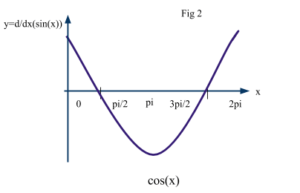# Differentiation of Trigonometric Functions

Contents

### Summary

Remember these derivatives of the trigonometric functions:

•$\frac { d }{ dx } \sin { (x) } \quad =\quad \cos { (x) }$
•$\frac { d }{ dx } \cos { (x) } \quad =\quad -\sin { (x) } \quad$
•$\frac { d }{ dx } \tan { (x) } \quad =\quad { sec }^{ 2 }(x)$
•$\frac { d }{ dx } \cot { (x) } \quad =\quad -co{ sec }^{ 2 }(x)$
•$\frac { d }{ dx } \sec { (x) } \quad =\quad \sec { (x) } \tan { (x) }$
•$\frac { d }{ dx } cosec(x)\quad =\quad -cosec(x)\cot { (x) }$

#### Differentiation of sin(x) and cos(x)

To begin with, we know that differentiation is a method to find the gradient of a curve.

Rule of differentiation is if$y\quad =\quad { x }^{ n }$, then$\frac { dy }{ dx } \quad =\quad n{ x }^{ n\quad -\quad 1 }$. However in this article we will focus entirely on differentiation of trigonometric functions.

Consider the graph of$y\quad =\quad \sin { (x) }$ in the range$0\quad \le \quad x\quad \le \quad 2\pi$. We draw tangents to the sin curve at the points where$x\quad =\quad 0,\quad x\quad =\quad \frac { \pi }{ 2 } ,\quad x\quad =\quad \pi ,\quad x\quad =\quad \frac { 3\pi }{ 2 } ,\quad x\quad =\quad 2\pi$ radians.x 0$\frac { \pi }{ 2 }$$\pi$$\frac { 3\pi }{ 2 }$$2\pi$ sin(x) 0 1 0 -1 0

We now plot the values of the gradients of these tangents and we obtain a graph of cos(x). As shown in Fig 2.Hence, this shows that derivative of sin(x) is cos(x). It can be written as:$\frac { d }{ dx } \sin { (x) } \quad =\quad \cos { (x) }$ when x in radians.

Similarly, we can find that:$\frac { d }{ dx } \cos { (x) } \quad =\quad -\sin { (x) } \quad$

#### Differentiation of tan(x)

Let’s assume$y\quad =\quad \tan { (x) }$,$y\quad =\quad \frac { \sin { (x) } }{ \cos { (x) } }$     using quotient rule$\frac { dy }{ dx } \quad =\quad \frac { \cos { (x)\cos { (x) } \quad -\quad \sin { (x) } (-\sin { (x) } ) } }{ { (\cos { (x) } ) }^{ 2 } }$$\frac { dy }{ dx } \quad =\quad \frac { { cos }^{ 2 }(x)\quad +\quad { sin }^{ 2 }(x) }{ { cos }^{ 2 }(x) }$$\frac { dy }{ dx } \quad =\quad \frac { 1 }{ { cos }^{ 2 }(x) } \quad =\quad { sec }^{ 2 }(x)$

#### Differentiation of sec(x)

Let’s assume:$y\quad =\quad \sec { (x) }$,$y\quad =\quad { cos }^{ -1 }(x)$$\frac { dy }{ dx } \quad =\quad -1({ cos }^{ -2 }(x))(-\sin { (x) } )$$\frac { dy }{ dx } \quad =\quad \frac { \sin { (x) } }{ { cos }^{ 2 }(x) } \quad =\quad (\frac { \sin { (x) } }{ \cos { (x) } } )(\frac { 1 }{ \cos { (x) } } )$$\frac { d }{ dx } sec(x)\quad =\quad sec(x)tan(x)$

#### Differentiation of cosec(x)

Assume$y\quad =\quad cosec(x)\quad =\quad \frac { 1 }{ \sin { (x) } } \quad =\quad { sin }^{ -1 }(x)$

Taking the derivative:$\frac { dy }{ dx } \quad =\quad -1({ sin }^{ -2 }(x))\cos { (x) }$$\frac { dy }{ dx } \quad =\quad \frac { -cos(x) }{ { sin }^{ 2 }(x) }$$\frac { dy }{ dx } \quad =\quad -(\frac { \cos { (x) } }{ \sin { (x) } } )(\frac { 1 }{ \sin { (x) } } )$$\frac { dy }{ dx } \quad =\quad -cot(x)cosec(x)$$\frac { d }{ dx } cosec(x)\quad =\quad -cot(x)cosec(x)$

Hence, to conclude derivatives of trigonometric functions are:$\frac { d }{ dx } \sin { (x) } \quad =\quad \cos { (x) }$$\frac { d }{ dx } \cos { (x) } \quad =\quad -\sin { (x) } \quad$$\frac { d }{ dx } \tan { (x) } \quad =\quad { sec }^{ 2 }(x)$$\frac { d }{ dx } \cot { (x) } \quad =\quad -co{ sec }^{ 2 }(x)$$\frac { d }{ dx } \sec { (x) } \quad =\quad \sec { (x) } \tan { (x) }$$\frac { d }{ dx } cosec(x)\quad =\quad -cosec(x)\cot { (x) }$

#### Example 1

Q. Differentiate${ sin }^{ 2 }(x)$ with respect to x

Solution:$y\quad =\quad { sin }^{ 2 }(x)$$\frac { dy }{ dx } \quad =\quad 2\sin { (x) } \quad \quad \frac { d }{ dx } sin(x)$$\quad =\quad 2sin(x)\quad cos(x)$   use the identity$\quad sin(2x)\quad =\quad 2sin(x)cos(x)$

Ans:$=\quad sin(2x)$